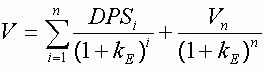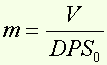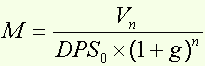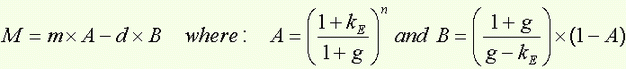The Bates formula

Around the formula...

The Bates formula establishes a relationship between the current P/E of a stock and its future P/E (in n years), given the Dividend Per Share (DPS) growth, the payout ratio and the required rate of return during the period.

The Bates formula is based on the formula used to determine the present value of cash flows on a given period (n years) :In this model, dividends (and therefore, dividends per share DPS), are expected to grow at a constant rate g, and the payout ratio d is also constant.

m being the current P/E :and M, the forecasted P/E in n years :We obtain :